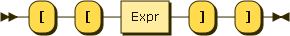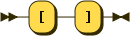### Array member selector

Figure 45. ArrayLookupArray lookup uses double square brackets.

Example 87. Array lookup

```[ "foo", "bar" ] []
```

Result (run with Zorba): bar

Since JSONiq expressions are composable, you can also use any expression for the left-hand side. You might need parentheses depending on the precedence.

Example 88. Array lookup after an object lookup

```{ field : [ "one",  { "foo" : "bar" } ] }.field[].foo
```

Result (run with Zorba): bar

The array lookup operator does an implicit mapping on the left-hand-side, i.e., it applies the lookup in turn on each item. Lookup on an array returns the item at that position in the array, or the empty sequence if there is none (position larger than size or smaller than 1). Lookup on any item which is not an array (objects and atomics) results in the empty sequence.

Example 89. Array lookup with an iteration on several arrays

```([ 1, 2, 3 ], [ 4, 5, 6 ])[]
```

Result (run with Zorba): 2 5

Example 90. Array lookup with an iteration on a collection

```collection("captains").series[]
```

Result (run with Zorba): The original series The next generation The next generation The next generation Entreprise Voyager

Example 91. Array lookup on a mixed sequence

```([ 1, 2, 3 ], [ 4, 5, 6 ], { "foo" : "bar" }, true)[]
```

Result (run with Zorba): 3 6

The expression inside the double-square brackets may be any expression. The result of evaluating this expression is cast to an integer. An error is raised if the cast fails.

Example 92. Array lookup with a right-hand-side expression

```[ "foo", "bar" ] [[ 1 + 1 ]]
```

Result (run with Zorba): bar

Figure 46. ArrayUnboxingYou can also extract all items from an array (i.e., as a sequence) with the [] syntax. The [] operator also implicitly iterates on the left-hand-side, returning the empty sequence for non-arrays.

Example 93. Extracting all items from an array

```[ "foo", "bar" ][]
```

Result (run with Zorba): foo bar

Example 94. Extracting all items from arrays in a mixed sequence

```([ "foo", "bar" ], { "foo" : "bar" }, true, [ 1, 2, 3 ] )[]
```

Result (run with Zorba): foo bar 1 2 3